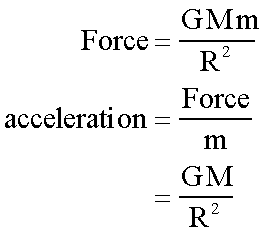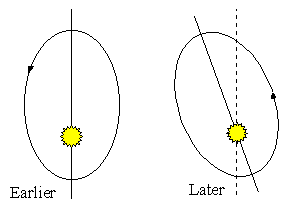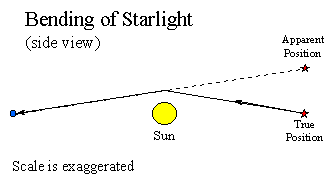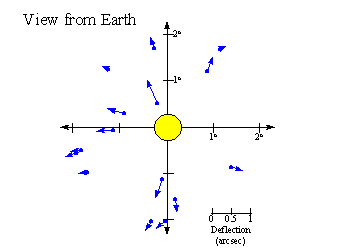Astronomy 162: Introduction to Stars, Galaxies, & the Universe Prof. Richard Pogge, MTWThF 9:30

# Lecture 32: Space, Time & Gravity General Relativity

Readings: Ch 24, section 24-2

## Key Ideas

General Relativity:
Modern Theory of Gravitation
Matter tells spacetime how to curve.
Curved spacetime tells matter how to move.

Tests of General Relativity:
Perihelion Precession of Mercury
Bending of Starlight near the Sun

## Newtonian Gravity

• Matter tells gravitation how to exert a Force.
• A Force tells matter how to accelerate.

A mass m is accelerated by another mass M:## Einstein's Discontents

Einstein had two objections to Newton's formulation of Gravity:
1. The force law (line 1) implies instantaneous knowledge of the distance, R, but information is only transmitted at the speed of light.

2. The same mass, m, appears in both the force and acceleration laws (lines 1 & 2), but disappears from the final acceleration (line 3). This seems to be coincidental.

## "I frame no hypothesis."

Newton could not explain what gravity was.
• He asserted that Gravity is an "action at a distance".
• He had no hypothesis for what "agent" communicates the gravitational force across empty space.
• People assumed that gravity worked as described by Newton, but didn't seem to worry about why it worked that way...

## The Principle of Equivalence

The first postulate of Special Relativity states:
• The laws of physics are the same for all uniformly moving observers.

In 1907, Einstein generalized this statement:

• The laws of physics are the same for any freely falling observer.

There is no distinction between gravitational and inertial accelerations.

For example, a person sealed in a closed box (no windows) drops an apple and watches it fall down with an acceleration of 1-g. There are no experiments that can be performed inside the boxes that will distinguish between two possibilities:

1. They and the box are at rest on the surface of the Earth, and the apple is accelerated downward by the Earth's gravity at 1-g.

2. They and the box are in free space accelerating upwards at 1-g by constantly burning rocket engines, and the apple appears to accelerate downwards because the floor of the box rises up to meet it.
Both views of the situation are equivalent and indistinguishable.

## General Relativity

Gravitation binds matter to matter.

But how does matter "know" that the other matter is "out there"?

The Goal: Generalize Relativity

• Special Relativity uses light to unify space & time into spacetime, but leaves matter separate.
• Need to unite matter & gravity through the agency of spacetime.

## Enter Geometry

Newton's laws lead to a geometric description of motion:

Least Action Principle:

• An object moves between two points along the path that takes the least amount of time.

Use geometry to describe the paths of objects moving through space.

But,

Einstein showed:

• Space & Time are relative, not absolute.
• Only spacetime is observer-independent.

Modified the Least Action Principle:

• An object moves along the shortest path between two points in spacetime.

Need to describe the geometry of spacetime.

## The Shortest Path...

On a flat surface:
• The shortest path between two points is a straight line.
• Parallel lines stay parallel always.

On a curved surface:

• The shortest path is a curved line.
• Lines that start parallel can converge or diverge at some distance away.

## Geometry the Unifier

In empty space, spacetime is flat:
• The least paths are straight lines.

Moving objects follow straight lines.

Newton would have said:

• "It moves in a straight line because it feels no external force to change its motion."

## Curved Spacetime

Matter curves the spacetime around it:
• The least paths are curved lines.
• More mass = Greater spacetime curvature.
• Closer = Greater spacetime curvature.

A freely falling object follows a curved path.

Newton would have said:

• "It feels a force deflecting it from a straight line path."

## A New Theory of Gravity

General Relativity may be summarized as follows:
Matter tells spacetime how to curve.
Curved spacetime tells matter how to move.

This replaces the Newtonian idea of a "force" with the curvature of spacetime as the agent of Gravity.

GR has so far withstood all experimental tests.

[Note: The summary statement above is due to physicist John Archibald Wheeler, who also coined the term "black hole" in the 1960s].

## The Precessing Orbit of Mercury

Mercury's orbit major axis precesses slowly by approximatesly 574 arcseconds/century:Einstein 1, Newton 0

Newtonian gravity:

• Predicts ~531 arcseconds/century.
• ~43 arcsec/century smaller than observed.

General Relativity:

• Spacetime curvature changes as Mercury gets closer to the sun on its orbit.
• Gives the orbit a little twist.
• This adds an extra 43 arcsec/century!!

## Bending of Starlight

Light travels on the shortest path through spacetime.

Prediction:

• Gravity bends light passing a massive object.
Confirmed: during the 1919 Total Solar Eclipse.Another manifestation of gravitational bending of light by massive objects is the phenomenon of "strong gravitational lensing". This is seen in some massive clusters of galaxies (and a few individual galaxies) with bright objects (galaxies or quasars) directly behind them. The Hubble Space Telescope has made some spectacular images of such gravitational lenses:

The huge masses of the galaxy clusters acts like a lumpy lens, distorting the images of more distant galaxies far behind them into luminous arcs. You can see an analogous effect by holding a lens at arm's length and looking at how distant objects are distorted as the lens passes between you and them.

## Whither Newton?

Newton's laws are approximations of GR.

Conditions:

• weak gravitational fields
• speeds much slower than the speed of light.

Newton's Laws:

• Work accurately in the "everyday" world.
• Are mathematically much simpler.
So we continue to use and teach Newtonian physics, only resorting to the more complex mathematics of General Relativity when a full treatment is required.

However, Relativity (both Special and General) do have real-world applications. For example, the GPS satellite navigation system.

Return to [ Unit 5 Index | Astronomy 162 Main Page ]
Updated: 2006 February 18